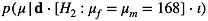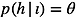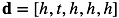top of page

#### A Beginners Guide to Bayesian Parameter Estimation (Measurement)

• What is measurement / parameter estimation?

• How are probabilities used in measurement?

• How is marginalization used in measurement?

• Can measurements be used to test hypotheses?What is measurement / parameter estimation?

Measurement is the most direct application of Bayes’ theorem

In the simplest such example, we have a series of coin-flip data (e.g,.                        ), and it takes the form:

where we are interested in measuring the value of the rate parameter,    .

Bayes’ theorem allows us to compute the probabilities of the various possible values of the rate parameter, and choose the parameter value most consistent with the data

• that is, it allows us to measure the value of

In a more typical example, such as wanting to measure a length using data from observations of a ruler, Bayes’ theorem takes the form:

where we are interested in measuring the value of the length parameter,   .

This equation tells us the probabilities of different values of the parameters    and    , based on having observed the dataset, d

• It therefore constitutes a measurement of those parameters, based on the data.

• because it allows us to estimate, or measure, the values of those parameters

The additional complication in this equation arises because there is a ‘nuisance parameter’,     , in the problem

• Nuisance parameters are dealt with via marginalization (the integral in the second example)

• Marginalization allows us to measure the    parameter, independent of the value of

How are probabilities used in measurement?

The posterior probability distribution over the rate parameter,              , is the output of Bayes’ theorem

• it allows us to determine which values are most likely to be the true value of the rate parameter, based on having observed the dataset d.

The process of computing these probabilities proceeds in two stages:

1) First, we assign a sampling distribution based on the error model representing coin flips. Coin-flips are represented mathematically as Bernoulli trials, in which each flip is assigned a constant probability of showing heads,                , and a complimentary probability of showing tails,                    .

This allows us to derive the likelihood function associated with the dataset,                        . The likelihood function tells us the sampling probabilities associated with this dataset for all values of the rate parameter:

where k represents the number of heads observed in our coin-flip dataset, and n is the total length of the flipping series.

2) The next stage requires us to assign probabilities to the rate parameter to represent our information about its possible values prior to having seen the coin-flip data.

• Prior to collecting data, we probably have some idea of what the values of the rate parameter are possible.

• If all values are possible, we might want to assign a uniform prior distribution:

,

• if we want to assign a transformation invariant, maximally uninformed prior distribution we would assign the Jeffreys prior:

• In the length measurement example, we would typically assign the Jeffreys priors:

and

based on the Gaussian likelihood:

The probabilities,                     , that are the output of Bayes’ theorem allow us to determine which values are most likely to be the true values of the parameters.

• The integral in Bayes’ theorem allows us to compute the posterior distribution over    , independent of the value of

• this is called 'marginalizing out' the nuisance parameter      from the problem

• marginalization is a consequence of the sum rule of probability theory

Based on our coin-flipping example, let’s go ahead and make the measurement.

We start with the prior distribution, which we will assign uniform probabilities:

nthetas=501;

thetas=linspace(0,1,nthetas);

logptheta=zeros(nthetas,1)/nthetas;

Next, we compute the likelihood function:

d=logical([1 0 1 1 1]);

logLtheta=sum(d)*log(thetas)+sum(~d)*log(1-thetas);

logptheta=logptheta+logLtheta;

and finally we plot the result (Fig. 1a):

figure; subplot(2,1,1)

plot(thetas,exp(logptheta),'k-')

plot(logpeakval([logptheta thetas]),'ko','MarkerFaceColor',[.1 .2 .4])

xlabel('rate'); ylabel('p(theta|data)')

• Notice we plot the peak of the posterior distribution (             ) and also the interval of the    -axis covering 95% of the mass of the posterior distribution.

• The range of the 95% confidence interval for your measurement is quite wide, covering more than half of the    -axis.

• To see how the 95% confidence interval will change as more data are acquired, we can increase the size of the dataset (while keeping the rate of heads constant in the dataset) by quadrupling the dataset to:                                                       .

• This new analysis (replacing d in the code above) yields the plot in Fig. 1b.

• We see that the larger dataset yields a much narrower confidence range

Can a measurement be used to test hypotheses?

Measurement and hypothesis testing are two distinct applications of Bayes’ theorem, and they are not interchangeable.

• Confusing the two is one of the basic logical errors of the foundations of classical statistical data analysis

• To get a taste of the issue, let’s take the length measurement example again, because this is the paradigm measurement scenario

• the great majority of other examples can be thought of as expansions of this simple measurement scenario

• In the measurement scenario, our prior information about the length was that it could take any value.

• This information was encoded in the prior probability distribution,

• This prior has no term for    , because any value of    has associated with it the same probability.

This is quite different from model selection, which is what Bayesians call hypothesis testing.

• Within Bayesian model selection, we define at least two distinct models.

• One case of model selection for two competing hypotheses predicting different lengths might be:

H1: The height of a child is equal to the height of the same-sex parent

H2: The height of a child is equal to the mean height of both parents

• These hypotheses make distinct predictions.

• For example, the average [female, male] height-pair is [161cm, 175cm] in the United States.

• Female children are predicted to be 161cm tall by H1 and 168cm tall by H2

• Male children are predicted to be 175cm tall by H1 and 168cm tall by H2

When we compare these hypotheses, we are (in part) comparing posteriors for the two models:

For H1 the posterior of interest is:

For H2 the posterior of interest is:

• This paints a picture quite distinct from the measurement scenario, because there is only a single possible value of height for male and female children under each of the two hypotheses, as dictated by the conditioning statements.

• For example, the normalized posterior probability that a child grows to be 168cm tall under H2 is            , regardless of the data.

• This is because it is the only possibility allowed under the hypothesis, H2: the hypothesis we are asserting in the conditioning statement of the second posterior.

• a similar statement holds for H1 (i.e., the probability of the predicted heights is unity)

• In contrast, the conditioning statements of the posterior for the measurement of height allowed all values.

• By allowing all values, or even just allowing a range of values that encompass the predictions of H1 and H2, we violate the background information of both of these models.

• H1 requires certain specific average heights, and a model that allows other heights runs counter to H1.

• The same is obviously true of H2

Measurement and hypothesis testing are distinct and mutually exclusive enterprises.

• Within classical statistical data analysis, confusing measurement and hypothesis testing contributes to one of its major logical flaws

• classical statistical hypothesis testing attempts to mimic the logically correct method of reasoning called 'proof by contradiction'

• this method requires that one observe outcomes that are impossible relative to the model’s predictions

• outcomes that are impossible under the null model only occur in extraordinary circumstances within classical statistics

• an example would be: observing a 'heads' outcome when flipping a coin that is hypothesized to be stamped with two 'tails'

• however, there are no impossible outcomes for Gaussian sampling (e.g., no length datum is an impossible sample to observe when the predicted mean sample is x =   )

• Gaussian sampling distributions are the most common sampling distribution used in classical and Bayesian data analysis

• and indeed there are special procedures called normality tests used to 'verify Gaussian sampling' in classical statistics.bottom of page